Home > A2C > Chapter 7 > Lesson 7.1.5 > Problem7-86

7-86.
1. Complete the area model shown below, then complete parts (a) through (c) below. Homework Help ✎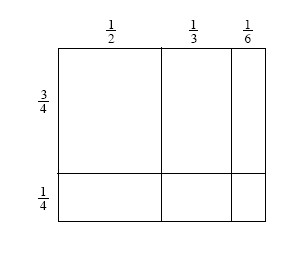1. What is the sum of the areas of each of the rectangles that makes up the larger one?

2. What are the length and width of the large rectangle?

3. Write a statement of the form l · w = Area as a sum for this rectangle.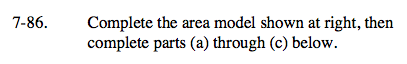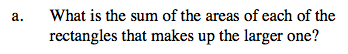Multiply the sides to find the area of the six smaller rectangles.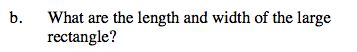Add all the areas of the six smaller rectangles.
Remember to find a common denominator.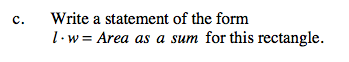See parts (a) and (b) for the length, width, and area.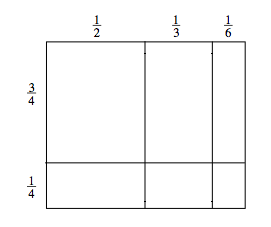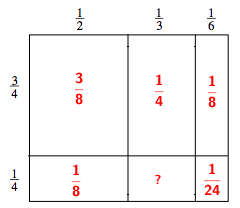Add the values above the large rectangle together for the width and then add the ones to the side for the height.

Both should equal 1.